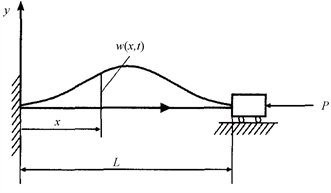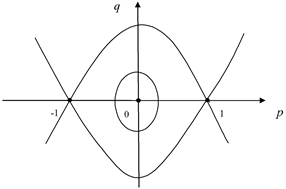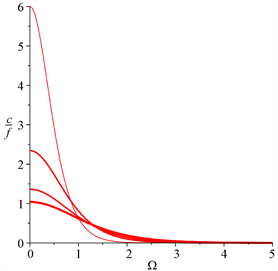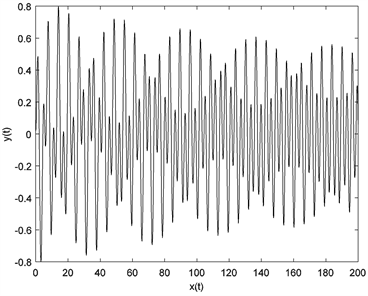# 轴向运动曲梁的次谐分岔和混沌Subharmonic Bifurcations and Chaos for the Buckled Beam at Axial Motion

DOI: 10.12677/AAM.2019.87149, PDF, HTML, XML, 下载: 337  浏览: 460  科研立项经费支持

Abstract: The subharmonic bifurcations and chaos for one kind of buckled beam model subjected to para-metric excitations are investigated. The critical curves separating the chaotic and non-chaotic re-gions are obtained by utilizing Melnikov method. The conditions for subharmonic bifurcations are also obtained. Numerical results are given, which verify the analytical ones.

1. 引言

2. 问题描述Figure 1. A buckled beam at axial motion

$\begin{array}{l}\stackrel{¨}{v}+{v}^{iv}+4{\text{π}}^{2}{v}^{″}-2{b}^{2}{\text{π}}^{3}\mathrm{cos}2\text{π}x{\int }_{0}^{1}{v}^{\prime }\mathrm{sin}2\text{π}x\text{d}x\\ =b{\text{π}}^{2}\mathrm{cos}2\text{π}x{\int }_{0}^{1}{{v}^{\prime }}^{2}\text{d}x+b\text{π}{v}^{″}{\int }_{0}^{1}\mathrm{sin}2\text{π}x\text{d}x+\frac{1}{2}{v}^{″}{\int }_{0}^{1}{{v}^{\prime }}^{2}\text{d}x-c\stackrel{˙}{v}+F\mathrm{cos}\Omega t\end{array}$ (1)

$x=0$$x=1$ 时， $v=0$${v}^{\prime }=0$

$\stackrel{¨}{q}+{\omega }^{2}q=-c\stackrel{˙}{q}+b{a}_{2}{q}^{2}+{a}_{3}{q}^{3}+f\mathrm{cos}\Omega t$ (2)

$\left\{\begin{array}{l}\stackrel{˙}{x}=y\\ \stackrel{˙}{y}=-{\omega }^{2}x+{a}_{3}{x}^{3}-\epsilon cy+\epsilon f\mathrm{cos}\Omega t\end{array}$ (3)

$\epsilon =0$ 时，系统(3)的未扰动系统为

$\left\{\begin{array}{l}\stackrel{˙}{x}=y\\ \stackrel{˙}{y}=-{\omega }^{2}x+{a}_{3}{x}^{3}\end{array}$ (4)

(4)是Hamiltonian系统，其Hamiltonian量为

$H=\frac{1}{2}{y}^{2}+\frac{{\omega }^{2}}{2}{x}^{2}-\frac{{a}_{3}}{4}{x}^{4}$ (5)

3. 系统的次谐分岔与混沌

${a}_{3}>0$ 的混沌行为

${a}_{3}>0$ 时，利用如下变换

$u=p\frac{\sqrt{{a}_{3}}}{\omega }$$t\to \frac{1}{\omega }t$ (6)

$\left\{\begin{array}{l}\stackrel{˙}{u}=v\\ \stackrel{˙}{v}=-u+{u}^{3}-\epsilon \stackrel{¯}{c}q+\epsilon \stackrel{¯}{f}\mathrm{cos}\left(\stackrel{¯}{\Omega }t\right)\end{array}$ (7)

$\left\{\begin{array}{l}\stackrel{˙}{u}=v\\ \stackrel{˙}{v}=u-{u}^{3}\end{array}$ (8)

$H\left(u,v\right)=\frac{1}{2}{v}^{2}+\frac{1}{2}{u}^{2}-\frac{1}{4}{u}^{4}$ (9)Figure 2. The phase portrait of system (8)

$\left\{\begin{array}{l}u\left(t\right)=±\mathrm{tanh}\left(\frac{\sqrt{2}}{2}t\right)\\ v\left(t\right)=±{\mathrm{sech}}^{2}{\frac{\sqrt{2}}{2}}^{2}\left(\frac{\sqrt{2}}{2}t\right)\end{array}$ (10)

$h=h\left(k\right)$ 为参数的周期轨道为

$\left\{\begin{array}{l}{u}_{k}\left(t\right)=\frac{\sqrt{2}k}{\sqrt{1+{k}^{2}}}sn\left(\frac{t}{\sqrt{1+{k}^{2}}},k\right)\\ {v}_{k}\left(t\right)=\frac{\sqrt{2}k}{1+{k}^{2}}cn\left(\frac{t}{\sqrt{1+{k}^{2}}},k\right)dn\left(\frac{t}{\sqrt{1+{k}^{2}}},k\right)\end{array}$ (11)

$M\left({t}_{0}\right)=-{\int }_{-\infty }^{+\infty }\stackrel{¯}{c}{v}^{2}\left(t\right)\text{d}t-{\int }_{-\infty }^{+\infty }\stackrel{¯}{f}v\left(t\right)\mathrm{cos}\stackrel{¯}{\Omega }\left(t+{t}_{0}\right)\text{d}t=-\stackrel{¯}{c}{J}_{0}-\stackrel{¯}{f}{J}_{1}\mathrm{cos}\stackrel{¯}{\Omega }{t}_{0}$ (12)

${J}_{0}=\frac{2\sqrt{2}}{3}$${J}_{1}=\sqrt{2}\text{π}\stackrel{¯}{\Omega }\mathrm{csch}\left(\frac{\sqrt{2}}{2}\text{π}\stackrel{¯}{\Omega }\right)$(13)

$\frac{c}{f}\le \frac{\sqrt{{a}_{3}}}{{\omega }^{2}}|\frac{{J}_{1}}{{J}_{0}}|$

$M\left({t}_{0}\right)$ 存在零点，系统发生混沌。取不同的 $\omega$ 值，比如 $\omega =0.5,1,1.5,2$ ，得到系统发生混沌的临界曲线，如图3所示。在曲线下方是发生混沌的区域，在曲线上方是非混沌区域。Figure 3. The critical curves for chaotic motions

4. 通向混沌的道路

$\begin{array}{c}{M}^{m/n}\left({t}_{0}\right)={\int }_{0}^{mT}\left(-\stackrel{¯}{c}\right){v}_{k}^{2}\left(t\right)\text{d}t+\stackrel{¯}{f}{\int }_{0}^{mT}{v}_{k}\left(t\right)\mathrm{cos}\stackrel{¯}{\Omega }\left(t+{t}_{0}\right)\text{d}t\\ =-\stackrel{¯}{c}{J}_{0}\left(m,n\right)+\stackrel{¯}{f}{J}_{1}\left(m,n\right)\mathrm{cos}\stackrel{¯}{\Omega }{t}_{0}\end{array}$ (14)

${J}_{0}\left(m,n\right)={\int }_{0}^{mT}{v}_{k}^{2}\left(t\right)\text{d}t=\frac{8n\left[\left({k}^{2}-1\right)K\left(k\right)+\left({k}^{2}+1\right)E\left(k\right)\right]}{3{\left({k}^{2}+1\right)}^{\frac{3}{2}}}$ (15)

${J}_{1}\left(m,n\right)={\int }_{0}^{mT}{v}_{k}\left(t\right)\mathrm{sin}\stackrel{¯}{\omega }t\text{d}t=\left\{\begin{array}{l}0,\text{}n\ne 1或m为偶数\\ \frac{{\text{π}}^{2}m}{\sqrt{2{k}^{2}-1}K\left(k\right)}\mathrm{csch}\left(\frac{\text{π}m{K}^{\prime }\left(k\right)}{2K\left(k\right)}\right),\text{}n=1或m为奇数\end{array}$ (16)

${K}^{\prime }\left(k\right)=K\left({k}^{\prime }\right)=K\left(\sqrt{1-{k}^{2}}\right)$$E\left(k\right)$ 为第二类椭圆积分。

$\frac{\stackrel{¯}{c}}{\stackrel{¯}{f}}<|\frac{{J}_{1}\left(m,n\right)}{{J}_{0}\left(m,n\right)}|\equiv {R}_{m}^{1}\left(\omega \right)$ (17)

5. 数值模拟Figure 4. The phase portrait of system (2) for $\omega =1$Figure 5. The phase portrait of system (2) for $\omega =2$

6. 结论

  Abou-Rayan, A.M., Nayfeh, A.H., Mook, D.T., et al. (1993) Nonlinear Response of a Parametrically Excited Buckled Beam. Non-linear Dynamics, 4, 499-525. https://doi.org/10.1007/BF00053693  Afaneh, A.A. and Ibrahim, R.A. (1993) Nonlinear Response of a Initially Buckled Beam with 1:1 Internal Resonance to Sinusoidal Excitation. Nonlinear Dynamics, 4, 547-571. https://doi.org/10.1007/BF00162232  Moon, F.C. and Shaw, S.W. (1983) Chaotic Vibration of a Beam with Nonlinear Boundary Conditions. Non-Linear Mechanics, 18, 465-477. https://doi.org/10.1016/0020-7462(83)90033-1  Moon, F.C. (1988) Experiments on Chaotic Motions of a Forced Nonlinear Oscillator. Stranger Attractors. Journal of Applied Mechanics, 55, 190-196.  Suire, G. and Cederbaum, G. (1995) Periodic and Chaotic Behavior of Viscoelastic Nonlinear Bars under Harmonic Excitations. International Journal of Mechanical Sciences, 7, 753-772. https://doi.org/10.1016/0020-7403(95)00006-J  Danida, D.B. (1994) Mathematical Models Used in Studying the Chaotic Vibration of Buckled Beam. Mechanics Research Communications, 21, 321-335. https://doi.org/10.1016/0093-6413(94)90091-4  Anantha, R.S., Sunkar, T.S. and Ganean, S. (1994) Bifurcation, Catastro-phes and Chaos in a Pre-Buckled Beam. International Journal of Non-Linear Mechanics, 29, 449-462. https://doi.org/10.1016/0020-7462(94)90014-0  Neukirch, S., Frelat, J., Goriely, A., et al. (2012) Vibrations of Post-Buckled Rods: The Singular Inextensible Limit. Journal of Sound and Vibration, 331, 704-720. https://doi.org/10.1016/j.jsv.2011.09.021  张年梅, 杨桂通. 非线性弹性梁的动态次谐分岔与混沌运动[J]. 非线性动力学学报, 1996(3): 265-274.  张年梅, 杨桂通. 非线性弹性梁中的混沌带现象[J]. 应用数学和力学, 2003, 24(5): 450-454.  Pinto, O.C. and Goncalves, P.B. (2002) Active Non-Linear Control of Buckling and Vibrations of a Flexible Buckled Beam. Chaos Solitons and Fractals, 14, 227-239. https://doi.org/10.1016/S0960-0779(01)00229-6  Oumarou, A.S., Nana Nbendjo, B.R. and Woafo, P. (2011) Appearance of Horseshoes Chaos on a Buckled Beam Controlled by Disseminated Couple Forces. Communication in Nonlinear Science Numerical Simulation, 16, 3212-3218. https://doi.org/10.1016/j.cnsns.2010.11.010